GeeksforGeeks App
Open AppBrowser
Continue

# Angle between Two Vectors Formula

A vector quantity is those physical quantities, having both magnitude and direction. When two vectors act on a particle, then resultant action on the particle will depend on the angle between those vectors. Hence it is important to know the angle between them.

Some properties of Vector for angle calculation

A vector is represented by an arrow parallel to the direction of the vector.

• A vector remains unchanged if it is transmitted parallel to itself.
• Two vectors having the same direction are parallel vectors.
• Two vectors having opposite directions are anti-parallel vectors.
• Two vectors having the same magnitude and direction are equal vectors.
• Two vectors having the same magnitude and the opposite direction are called negative vectors.

### Dot Product

It is also known as the scalar product of vectors. It has only magnitude but no direction.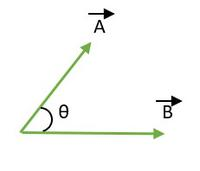Two vector A and B

Then Dot product of A and B is given by,= |A| |B| cosθ.

Special Cases

• When angle between vectors are 0 degree.

That is θ = 0°

⇒ |A| |B| cosθ.

⇒ |A| |B| cos0°

⇒ |A| |B| [cos0° = 1]

• When angle between vectors are 180 degree.

⇒ |A| |B| cosθ.

⇒ |A| |B| cos180°

⇒ – |A| |B| [cos180° = -1]

• When angle between vectors are 90 degree.

⇒ |A| |B| cosθ.

⇒ |A| |B| cos90°

⇒ |A| |B| × 0 [cos90° = 0]

⇒ 0.

### Formula for angle between two Vectors

The cosine of the angle between two vectors is equal to the sum of the product of the individual constituents of the two vectors, divided by the product of the magnitude of the two vectors.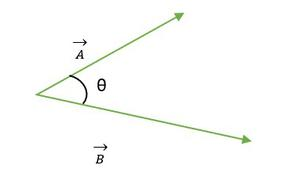Two Vectors A and B=| A | | B | cosθ.

cosθ=θ= cos-1In cartesian Form,

A = Axi + Ayj + Azk

B= Bxi + Byj + Bz

cosθ =Properties of Dot product

• Dot product is commutative.• Dot product is Distributive.In physics by convection, angle between two vectors lies between 0 ≤ θ ≤ 180. When the tails or heads of both the vectors coincide, then the angle between vectors is calculated.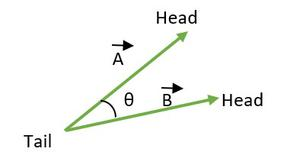Tail Coincide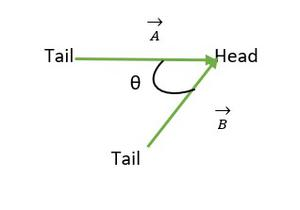### Sample Problems

Question 1: Find the angle between vectors (If they form an equilateral triangle)

• a and b vectors
• b and c vectors
• a and c vectors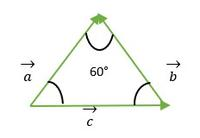Equilateral Triangle formed by a, b, c vector

Solution:

• a and b vectors

For vector a and b, head of both the vectors coincide with each other, hence angle between a and b vector is same as the angle between two sides of equilateral triangle = 60°.

• b and c vectors:

From the above figure, we see that head or tail of the b and c vector does not coincide with each other.

So, by using the property- A vector remains unchanged if it is transmitted parallel to itself.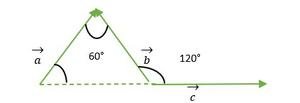Vector c is shifted parallel to itself

Now we see the tail of vectors b and c are coincide with each other, therefore is the same as the exterior angle make with an equilateral triangle = 120°.

• a and c vectorsThe tail of a and c coincide

For vectors a and c, the tail of both the vectors coincide with each other, hence the angle between the a and c vector is the same as the angle between two sides of the equilateral triangle = 60°.

Question 2: Find angles between vectors if they form an isosceles right-angle triangle.

• a and b vector
• b and c vector
• a and c vectors

Solution:

• a and b vector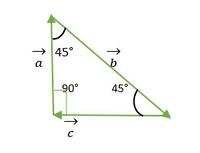Right angle Isosceles Triangle

From the above figure, we see that head or tail of a and b vector does not coincide with each other. So, by using the property- A vector remains unchanged if it is transmitted parallel to itself.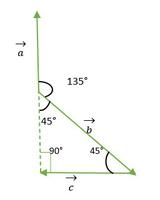a vector is shifted parallel to itself

Now, a and b vectors tails coincide with each other and make an angle the same as the exterior angle of a right angle isosceles triangle = 135°.

• b and c vectorRight angle Isosceles Triangle

From the above figure, b and c vector head or tails does not coincide with each other. So, by using the property, a vector remains unchanged if it is transmitted parallel to itself.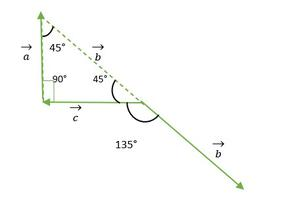b vector is shifted parallel to itself

Now, b and c vectors tails coincide with each other and make an angle the same as the exterior angle of a right angle isosceles triangle = 135°.

• a and c vectorsRight angle Isosceles Triangle

From the above figure, a and c vector head or tails do not coincide with each other. So, by using the property- A vector remains unchanged if it is transmitted parallel to itself.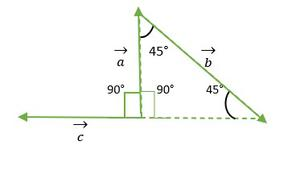c vector is moved parallel to itself

Now, a and c vectors tails coincide with each other and make an angle the same as the right angle of isosceles triangle = 90°.

Question 3: Find the angle between the vectors A = i + j + k and vector B = -2i – 2j – 2k.

Solution:

From the formula,

A = Axi + Ayj + Azk

B= Bxi + Byj + Bzk

cosθ=Here in the Given question,

A= i + j + k.

B= -2i -2j -2k.

Substituting the values in the formula

⇒ cosθ =⇒ cosθ =⇒ cosθ =⇒ cosθ =⇒ cosθ = -6/6

⇒ cosθ= -1

⇒ θ = 180°.

Question 4: Find angle between vector A = 3i + 4j and B = 2i + j

Solution:

A = Axi + Ayj + Azk

B = Bxi + Byj + Bzk

cosθ =Here Given,

A= 3i + 4j + 0k

B= 2i + j + 0k

Substituting the values in the formula,

⇒ cosθ =⇒ cosθ =⇒ cosθ =⇒ cosθ =⇒ θ = cos-1 ()

⇒ θ = cos-1 ()

Question 5: Find the angle between vector A = i + j and Vector B = j + k.

Solution:

From the formula,

A = Axi + Ayj + Azk

B = Bxi + Byj + Bzk

cosθ =Here in the Given question,

⇒ A = i + j

⇒ B = j + k

⇒ cosθ =⇒ cosθ =⇒ cosθ =.

⇒ θ = cos-1 (1/2)

⇒ θ = 60°.

My Personal Notes arrow_drop_up
Related Tutorials